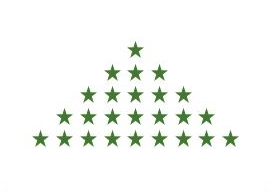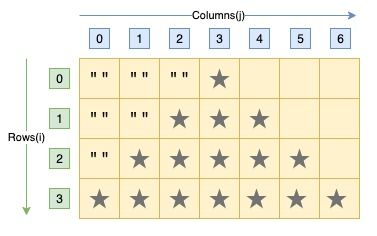# C Program to Print Pyramid Star Pattern

Here is a C program to print an equilateral triangle star pattern of n rows using loops.

For an equilateral triangle pyramid star pattern of 5 rows. Program's output should be:#### Required Knowledge

Algorithm to print pyramid star pattern using loop
In this program, we are printing a pyramid star pattern in which ith row contains (2*i + 1) space separated stars. The index of rows and columns starts from 0.
• We first take the number of rows(R) in the pattern as input from user using scanf function.
• One iteration of outer for loop will print a row of pyramid(from i = 0 to R - 1).
• For jth row of pyramid, the inner for loop first prints (R - i - 1) spaces for every line and then nested while loop prints (2*j + 1) stars.

Here is the matrix representation of the pyramid star pattern. The row numbers are represented by i whereas column numbers are represented by j.## C program to print pyramid star pattern

```#include<stdio.h>

int main() {
int i, j, rows, star = 0;
printf("Enter the number of rows\n");
scanf("%d", &rows);

/* printing one row in every iteration */
for (i = 0; i < rows; i++) {
/* Printing spaces */
for (j = 0; j <= (rows - i - 1); j++) {
printf(" ");
}
/* Printing stars */
while (star != (2 * i + 1)) {
printf("*");
star++;;
}
star = 0;
/* move to next row */
printf("\n");
}
return 0;
}
```
Output
```Enter the number of rows
6
*
***
*****
*******
*********
***********
```

## C program to print pyramid star pattern using single for loop

```#include<stdio.h>

int main(){
char *str="*****************";
int i,j, rows;

printf("Enter the number of rows\n");
scanf("%d", &rows);

for(i=0;i<rows;i++){
printf("%*.*s\n",rows+i, 2*i+1, str);
}
return 0;
}
```
Output
```Enter the number of rows
6
*
***
*****
*******
*********
***********
```

Related Topics
 C program reversed pyramid star pattern C program hollow pyramid star pattern C program hollow diamond star pattern C program right triangle star pattern C program hollow square star pattern C program heart shape star pattern C program natural number triangle pattern C program palindrome triangle pattern C program same row element triangle pattern List of all C pattern programs